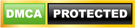Blog

# Understanding Data Parallelism in MapReduce

• ###### | 2306 Ratings

In order to understand the goals of MapReduce, it is important to realize for which scenarios MapReduce is optimized. The MapReduce programming model is created for processing data which requires “DATA PARALLELISM”, the ability to compute multiple independent operations in any order (King). In parallel processing, commutative operations are operations where the order of execution does not matter to the results of the equation. Commutativity can apply to complex operations and even processes, as long as they don’t manipulate the same memory. For example, in the figure below, as long as foo(a) and bar(b) don’t manipulate the same variable, they can occur in parallel in different threads. However, the write operation must wait for both foo() and bar() to complete. The figure below illustrates a dependency graph between foo(a), bar(a) and the write command.Figure 1 – Parallelism Dependency Graph

One of the goals of parallelism is identifying the logical “tasks” or units which can be run in parallel as threads. Parallel programming techniques require developers to implement dependency graphs, which can become much more as the amount of shared information and sequence of operations increases. Techniques such as locks and barriers, critical sections, semaphores, monitors, RPC and rendezvous have been proposed to aid in the design of multi threaded and distributed. In Parallel and Distributed processing, intelligent task design attempts to eliminate as many synchronization points as possible, but some will still be required. Patterns such as “Master/Worker” and “Producer/Consumer” are different patterns that developers can use to implement parallel thread processing.

MapReduce provides a programming model which abstracts many of the aforementioned complexities of parallel processing from the software engineer. The MapReduce implementation performs much of the “wiring” associated with parallel processing, leaving the developer to implement relatively simple methods. The use of MapReduce does come with some constraints, making it less appropriate for some tasks. MapReduce models are optimized for tasks where a large number of key*value input lists must be processed somewhat independently. MapReduce map() method must be commutative, in order for the MapReduce implementation to make use of parallelization. MapReduce enables the parallelization across hundreds and even thousands of CPU’s.

### List of Other Big Data Courses: